#DAY 3
4

## Line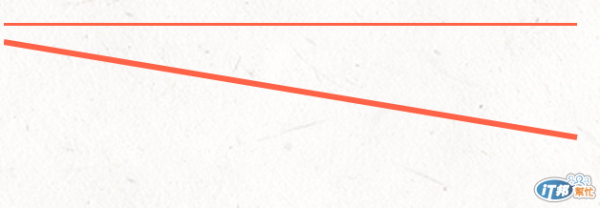<svg width="100%" height="100">
<line x1="0" y1="0" x2="300" y2="0" style="stroke: Tomato;stroke-width: 3px"/>
<line x1="0" y1="10" x2="300" y2="60" x3="0" y3="80" style="stroke: Tomato; stroke-width: 3px"/>
</svg>

## Polyline

Polyline我查到的中文翻譯是折線，它主要是繪製多點線段。來源...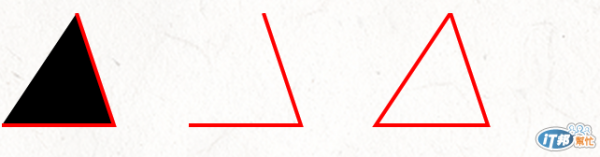<svg width="100%" height="100">
<polyline points="40,0 60,60 0,60" style=" stroke:red; stroke-width:2"/>
<polyline points="140,0 160,60 100,60" style="fill:none; stroke:red; stroke-width:2"/>
<polyline points="240,0 260,60 200,60 240,0" style="fill:none; stroke:red; stroke-width:2"/>
</svg>

Polyline開始類似Illustrator的鋼筆工具(但還不能繪製弧線)，在線段之間還能填入色彩，但特別要注意的是他並不是自動封閉線段。

## Polygon

Polygon中文翻譯為多邊形，用來繪製不少於3個邊的圖形，使用方式和Polyline完全相同。<svg width="100%" height="100">
<polygon points="40,0 60,60 0,60" style=" stroke:red; stroke-width:2"/>
<polygon points="140,0 160,60 100,60" style="fill:none; stroke:red; stroke-width:2"/>
<polygon points="240,0 260,60 200,60 240,0" style="fill:none; stroke:red; stroke-width:2"/>
</svg>

## 地獄般的Path

SVG <path>是相當有難度的一個章節，每次打開到這個章節，都會想要關掉網頁。它的難度就我目前看來，就是用鍵盤畫貝茲曲線吧...。

http://tutorials.jenkov.com/svg/path-element.html

https://developer.mozilla.org/en-US/docs/Web/SVG/Tutorial/Paths

M = moveto

L = lineto

H = horizontal lineto

V = vertical lineto

C = curveto

S = smooth curveto

T = smooth quadratic Bézier curveto

A = elliptical Arc

Z = closepath

Example M、L

M、L使用方法：

M x y
L x y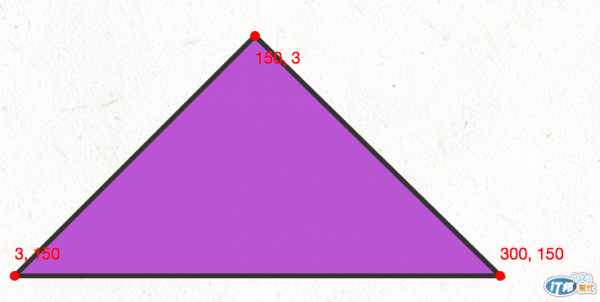<svg width="100%" height="200">
<path d="M150 3 L300 150 L3 150 Z" style=" stroke: #333; stroke-width:3; fill: MediumOrchid"/>
<!-- 硬派Circles! -->
<circle cx="150" cy="3" r="3" fill="red"/>
<text x="150" y="20" fill="red">150, 3</text>
<circle cx="300" cy="150" r="3" fill="red"/>
<text x="300" y="140" fill="red">300, 150</text>
<circle cx="3" cy="150" r="3" fill="red"/>
<text x="3" y="140" fill="red">3, 150</text>
</svg>

## Example H、V

H、V使用方法：

M x
V y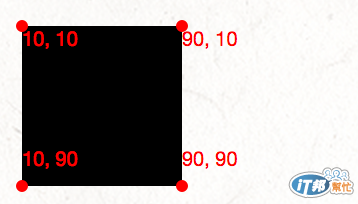<svg width="100%" height="100">
<path d="M10 10 H 90 V 90 H 10 L 10 10" fill="black"/>
<!-- 硬派Circles! -->
<circle cx="10" cy="10" r="3" fill="red"/>
<text x="10" y="20" fill="red">10, 10</text>
<circle cx="10" cy="90" r="3" fill="red"/>
<text x="10" y="80" fill="red">10, 90</text>
<circle cx="90" cy="90" r="3" fill="red"/>
<text x="90" y="80" fill="red">90, 90</text>
<circle cx="90" cy="10" r="3" fill="red"/>
<text x="90" y="20" fill="red">90, 10</text>
</svg>

## Example C 貝茲曲線

C使用方法：

C x1 y1, x2 y2, x y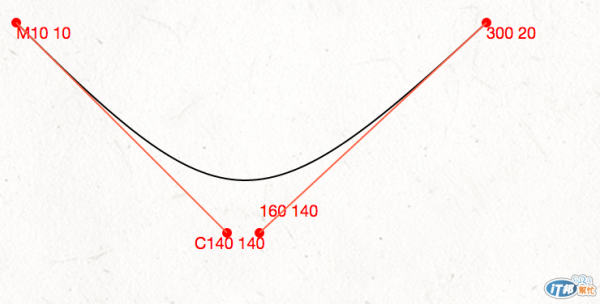<svg width="100%" height="200px">
<path d="M10 10 C140 140,160 140,300 10" stroke="black" fill="none"/>
<!-- 硬派Circles! -->
<circle cx="10" cy="10" r="3" fill="red"/>
<text x="10" y="20" fill="red">M10 10</text>
<circle cx="140" cy="140" r="3" fill="red"/>
<text x="120" y="150" fill="red">C140 140</text>
<circle cx="160" cy="140" r="3" fill="red"/>
<text x="160" y="130" fill="red">160 140</text>
<circle cx="300" cy="10" r="3" fill="red"/>
<text x="300" y="20" fill="red">300 20</text>
<line x1="10" y1="10" x2="140" y2="140" style="stroke: Tomato;"/>
<line x1="160" y1="140" x2="300" y2="10" style="stroke: Tomato;"/>
</svg>

## Example S

s使用方法：

S x2 y2, x y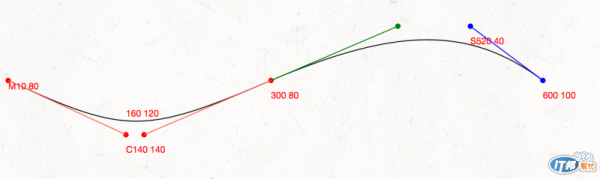<svg width="100%" height="200px">
<path d="M10 80 C140 140,160 140 , 300 80 S 520 20,600 80" stroke="black" fill="none"/>
<!-- 硬派Circles! -->
<circle cx="10" cy="80" r="3" fill="red"/>
<text x="10" y="90" fill="red">M10 80</text>
<circle cx="140" cy="140" r="3" fill="red"/>
<text x="140" y="160" fill="red">C140 140</text>
<circle cx="160" cy="140" r="3" fill="red"/>
<text x="140" y="120" fill="red">160 120</text>
<circle cx="300" cy="80" r="3" fill="red"/>
<text x="300" y="100" fill="red">300 80</text>
<circle cx="520" cy="20" r="3" fill="blue"/>
<text x="520" y="40" fill="blue">S520 40</text>
<circle cx="600" cy="80" r="3" fill="blue"/>
<text x="600" y="100" fill="blue">600 100</text>
<line x1="10" y1="80" x2="140" y2="140" style="stroke: Tomato;"/>
<line x1="160" y1="140" x2="300" y2="80" style="stroke: Tomato;"/>
<line x1="520" y1="20" x2="600" y2="80" style="stroke: blue;"/>
<circle cx="440" cy="20" r="3" fill="green"/>
<line x1="300" y1="80" x2="440" y2="20" style="stroke: green;"/>
</svg>

## Example Q T

Q、T使用方法：

Q x1 y1, x y
T x y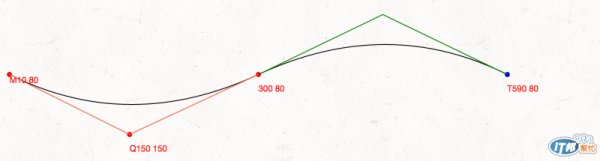<svg width="100%" height="200px">
<path d="M10 80 Q150 150, 300 80" stroke="black" fill="none"/>
<!-- 硬派Circles! -->
<circle cx="10" cy="80" r="3" fill="red"/>
<text x="10" y="90" fill="red">M10 80</text>
<circle cx="150" cy="150" r="3" fill="red"/>
<text x="150" y="170" fill="red">Q150 150</text>
<circle cx="300" cy="80" r="3" fill="red"/>
<text x="300" y="100" fill="red">300 80</text>
<line x1="10" y1="80" x2="150" y2="150" style="stroke: Tomato;"/>
<line x1="300" y1="80" x2="150" y2="150" style="stroke: Tomato;"/>

</svg>

## 小結

SVG 與 D3.js30

### 3 則留言

0

"我也試試看能不能三點，寫了x3、y3都是沒用的(白目)。"